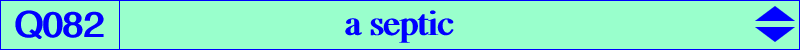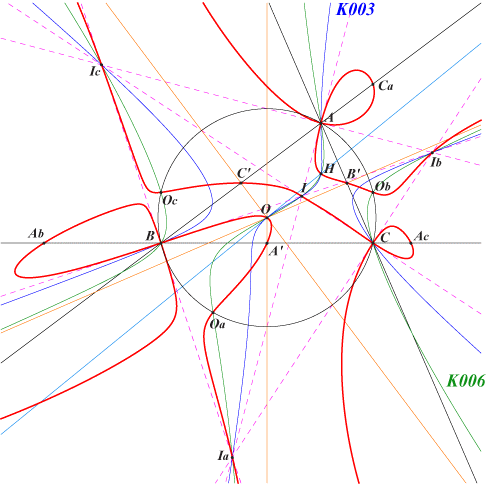too complicated to be written here. Click on the link to download a text file.X(1), X(3), X(4) excenters midpoints antipodes of A, B, C other points belowDenote by PaPbPc the pedal triangle of point P and by T1, T2, T3, T4, T5, T6 the areas of triangles PBPa, PPaC, PCPb, PPbA, PAPc, PPcB respectively. Let M1 and M2 be one of the means of T1, T3, T5 and T2, T4, T6 respectively. We seek the locus L of P such that M1 = M2. When the means are : arithmetic, L is the Stammler rectangular hyperbola, geometric, L is the Darboux cubic K006, quadratic, L is the pedalsix square cubic K100, harmonic, L is the septic Q082. Recall that the McCay cubic K003 is the locus of P such that T1*T3+T3*T5+T5*T1 = T2*T4+T4*T6+T6*T2. Q082 contains the 24 following points : A, B, C which are nodes with nodal tangents the internal and external tangents, A', B', C' midpoints of ABC, the in/excenters of ABC, the circumcenter O and the orthocenter H, the antipodes Oa, Ob, Oc of A, B, C on the circumcircle with tangents passing through H, the intersections of Ab, Ac of BC with the perpendiculars at A to AC, AB and four other points Bc, Ba, Ca, Cb similarly, the infinite points of the perpendicular bisectors of the sides of ABC, these bisectors being three real asymptotes of the septic. The 21 common points of the Darboux cubic and Q082 are clearly identified.